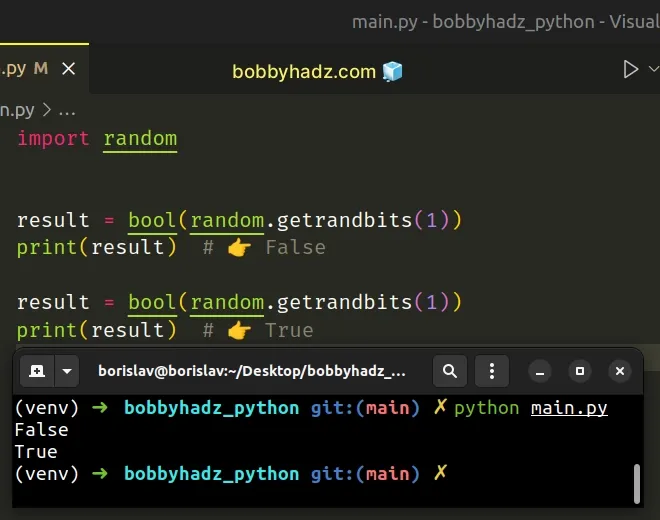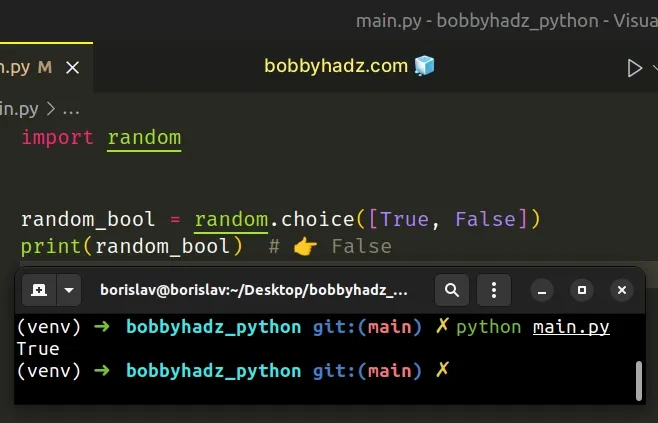# Generate random Boolean (True or False) values in PythonLast updated: Feb 22, 2023
4 min## #Generate a random Boolean using random.getrandbits()

To generate a random boolean value:

1. Use the `random.getrandbits()` method to get an integer with 1 random bit.
2. Use the `bool()` class to convert the integer to a boolean value.
main.py
```Copied!```import random

result = bool(random.getrandbits(1))
print(result)  # 👉️ False

result = bool(random.getrandbits(1))
print(result)  # 👉️ True
``````The random.getrandbits method returns a non-negative Python integer with `k` random bits.

We set the `k` argument to `1`, so the only possible values the method returns are `0` and `1`.

main.py
```Copied!```import random

print(random.getrandbits(1))  # 👉️ 1
print(random.getrandbits(1))  # 👉️ 0
print(random.getrandbits(1))  # 👉️ 0
``````

The last step is to use the bool() class to convert the integer to a boolean.

main.py
```Copied!```import random

result = bool(random.getrandbits(1))
print(result)  # 👉️ False

result = bool(random.getrandbits(1))
print(result)  # 👉️ True
``````

Converting `0` to a boolean returns `False` and converting `1` to a boolean returns `True`.

main.py
```Copied!```print(bool(0))  # 👉️ False
print(bool(1))  # 👉️ True
``````

## #Generate a random Boolean using random.choice()

You can also use the `random.choice()` method to generate a random boolean, but the method is a bit less performant.

main.py
```Copied!```import random

random_bool = random.choice([True, False])
print(random_bool)  # 👉️ False
``````The random.choice method takes a sequence and returns a random element from the non-empty sequence.

## #Generate a random Boolean using random.random()

You can also use the `random.random()` method to generate a random boolean.

main.py
```Copied!```import random

random_bool = random.random() > 0.5

print(random_bool)
``````

The random.random number returns the next random floating-point number in the range `[0.0,1.0)`.

The condition returns the result of checking if the generated number is greater than `0.5`.

## #Generate a random Boolean using random.randint()

You can also use the `random.randint()` method to generate random boolean values.

main.py
```Copied!```import random

random_bool = bool(random.randint(0, 1))

print(random_bool) # 👉️ True
``````

The random.randint function takes 2 numbers - `a` and `b` as parameters and returns a random integer in the range.

Note that the range is inclusive - meaning both `a` and `b` can be returned.

## #Generate a random Boolean based on probability

If you need to generate a random boolean value based on probability, use the `random.random()` method.

1. Use the `random.random()` method to get a random float from `0` to `1`.
2. Check if the generated value is less than the probability.
3. For example, `random.random() < 0.75` returns `True` 75% of the time.
main.py
```Copied!```import random

def bool_based_on_probability(probability=0.5):
return random.random() < probability

# 👇️ 75% probability to return True
result = bool_based_on_probability(0.75)
print(result)  # 👉️ True

# 👇️ 25% probability to return True
result = bool_based_on_probability(0.25)
print(result)  # 👉️ False
``````

The random.random number returns the next random floating-point number in the range `[0.0,1.0)`.

The method could return `0`, but it could never return `1`.

Comparing the two floating-point numbers returns a boolean value.

For example, if you set the `probability` argument to `0.75`, the function has a `75%` chance to return `True`.

If you set the argument to `1`, the function will always return `True`.

## #Generate a list of random boolean values using random.choices()

Use the `random.choices()` method to generate a list of multiple random boolean values.

The `random.choices()` method returns a `k` sized list of elements chosen from the iterable.

main.py
```Copied!```import random

random_boolean_list = random.choices([True, False], k=3)
print(random_boolean_list)  # 👉️ [True, True, False]

# 👇️ with probability (specify weights)
random_boolean_list = random.choices(
[True, False],
k=3,
weights=[75, 25]
)
print(random_boolean_list)  # 👉️ [True, True, False]
``````

The random.choices method returns a `k` sized list of elements chosen from the provided iterable with replacement.

main.py
```Copied!```import random

a_list = ['bobby', 'hadz', '.', 'com']

result = random.choices(a_list, k=3)
``````
With replacement basically means that the same element can be returned multiple times.

## #Generate a list of random boolean values based on probability

If you need to return the boolean values based on probability, set the `weights` argument in the call to `random.choices()`.

main.py
```Copied!```import random

random_boolean_list = random.choices(
[True, False],
k=3,
weights=[75, 25]
)
print(random_boolean_list)  # 👉️ [True, True, False]
``````

The call to the method has a 75% chance to return a `True` value and a 25% chance to return `False`.

## #Generate a random boolean value using numpy.random.rand()

You can also use the `numpy.random.rand()` method to generate a random boolean value.

main.py
```Copied!```import numpy as np

bool_array = np.random.rand() > 0.5

print(bool_array)  # 👉️ False
``````

Make sure you have the NumPy module installed to be able to run the code sample.

shell
```Copied!```pip install numpy

pip3 install numpy
``````

The numpy.random.rand method returns a single Python float from `0` to `1`.

main.py
```Copied!```import numpy as np

print(np.random.rand()) # 👉️ 0.400755652315017
``````

We compare the float to `0.5` to get a boolean result.

## #Generate an array of random booleans using numpy.random.randint()

You can also use the `random.randint()` method from the NumPy module to generate an array of random booleans.

main.py
```Copied!```import numpy as np

bool_array = np.random.randint(2, size=8) > 0.5

# [ True  True False False  True False  True False]
print(bool_array)
``````

Make sure you have the NumPy module installed to be able to run the code sample.

shell
```Copied!```pip install numpy

pip3 install numpy
``````

The numpy.random.randint() method returns random integers from low (inclusive) to high (exclusive).

main.py
```Copied!```import numpy as np

bool_array = np.random.randint(2, size=8)

# [0 0 1 1 1 0 1 0]
print(bool_array)
``````

We simply compare the array to `0.5` to get an array of random boolean values.

main.py
```Copied!```import numpy as np

bool_array = np.random.randint(2, size=8) > 0.5

# [ True  True False False  True False  True False]
print(bool_array)
``````